To change the display dates for the x:axis in a Bar/Line chart, you normally select the Properties panel, change the format to Date/Time, and select the date format you want to display on the x:axis.  However, you might have noticed though that you cannot select quarters when displaying your dates on the x:axis.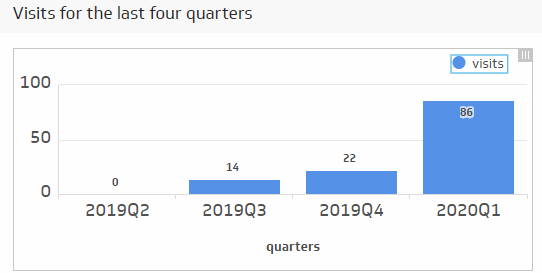To do this, you can make use of the DATE_UNITVALUE function.  In this example, we display the number of visits for the last four quarters.

The X:Axis

To get the year to display on the x:axis, you can use a formula like this one.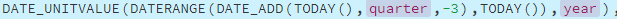In this formula, we use the DATERANGE function to specify a start date and end date.

The DATE_ADD function allows us to specify a start date of 4 quarters ago (-3).

The second TODAY function is allowing us to specify an end date of today’s date.

This formula will return the years.

We can use a similar formula to return the quarters.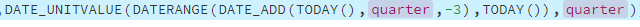And then to join the year and the quarter formulas together, we can use the CONCAT function.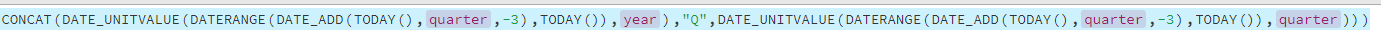Notice, we have also added the letter Q before the quarter number using the CONCAT function.

Finally, use the GROUP function to return one unique instance of the year and quarter.

The final formula for our x:axis looks like this: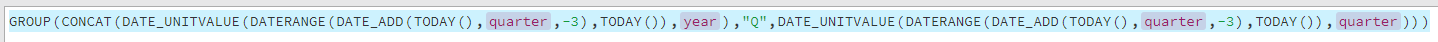Check that the format for the x:axis in the Properties panel is set to text.

The Series

Now that you have the x:axis set up to display the last four quarters, you will want to set up your series to plot your data from your data source.

You can do this using the LOOKUP function.

The LOOKUP function has three parameters and is a good function to know when it comes to aligning your data with the x:axis.

For the first parameter, we can simply type & and point to our x:axis. This is known as a Results Reference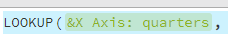For the second parameter, we can copy our x:axis formula and adjust it so it takes in the columns in our data source.

For example,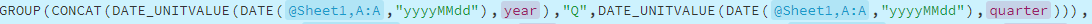You will see we still have our DATE_UNITVALUE function, but now we are referencing column A in our data source for our dates.

The DATE_UNITVALUE function requires that our dates are epoch numbers, so we need to use the DATE function to specify the date format of our dates to do this conversion.

For the third parameter, we can copy this formula and change the GROUP to the GROUPBY function and use the GROUPBY’s second parameter to point to the column in our data source that we want to measure (column B).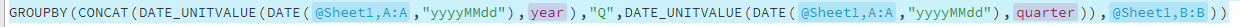The final formula for our series looks like this: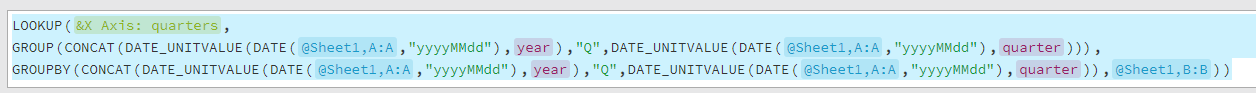Check that the format for the series in the Properties panel is set to sum.  It should be because the GROUPBY function by default will sum all values.

If you need any further explanation of the functions used in this example Klip, check out our Klipfolio functions article.